Courses

# Thermodynamic Relations Notes | EduRev

## Mechanical Engineering : Thermodynamic Relations Notes | EduRev

The document Thermodynamic Relations Notes | EduRev is a part of the Mechanical Engineering Course Thermodynamics.
All you need of Mechanical Engineering at this link: Mechanical Engineering

Introduction

The properties such as temperature pressure, volume, and mass can be calculated directly.
The substance's properties such as density (ρ) and specific volume(v) can be estimated using some simple relations.
However, energy, enthalpy (h), and entropy (s) are not very easy to determine because they are not measurable or can not be easily expressed in terms of measurable properties through some simple relations.

1. Maxwell’s Equations

These are the set of equations that establish the relation between the partial derivatives of properties P, V, T, and S of a simple compressible system.
dU = TdS – PdV
dH = TdS + VdP

Helmholtz function
F = U – TS (availability of closed system)

Gibb’s function
G = H – TS (availability of open system)
For all real processes, the value of the Helmholtz function & Gibbs function decreases & attains a min value at equilibrium.
Hence Four Maxwell’s relations are,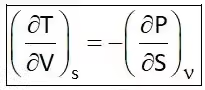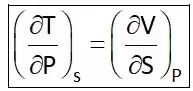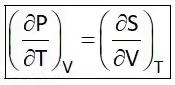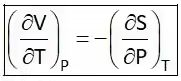Coefficient of Volume Expansion (β)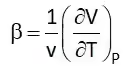It is the rate of change of volume with respect to temperature at constant pressure. Isothermal Compressibility (Kt)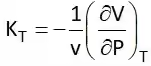It shows the rate of change of volume with respect to pressure at a constant temperature or at isothermal conditions.

2. T-dS Equation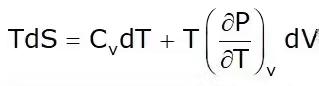This is known as the first Tds equation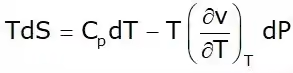This is known as the second TdS equation.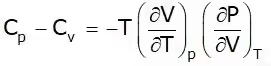T = Positive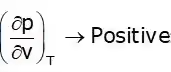Cp – Cv = +ve
Cp > Cv

3. Energy Equation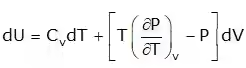Joule Thompson Coefficient (µ)
When a fluid passes through the porous plug, capillary tube, or valve, its pressure decreases. The throttling process is isenthalpic in nature. The temperature behavior of the fluid during throttling is described by the joule Thompson coefficient(µ),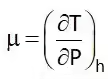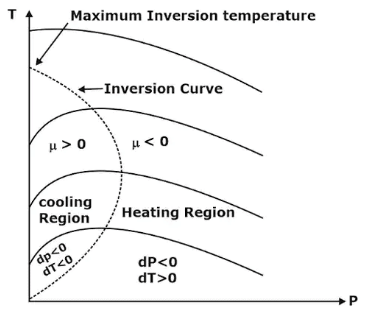Fig:1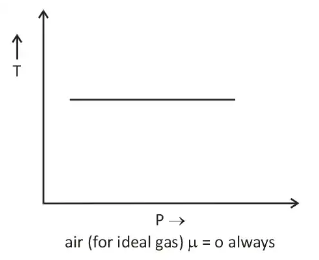Fig:2

Important points:

• Joule Thomson coefficient is +ve in cooling region i.e slope of isenthalpic curve on T – P diagram is +ve in the cooling region,
• μ is –ve in heating region i.e the slope of isenthalpic curve on T – P diagram is –ve in the heating region,
• There is nothing as a heating or cooling region for an ideal gas & the value of the joule Thomson coefficient is zero everywhere.

4. Clausius Clapeyron Equations

Clausius Clapeyron equations is a relationship between saturation pressure, temperature, and enthalpy of vaporization and the specific volume of two phases involved. This equation helps in the calculations of properties in two-phase regions.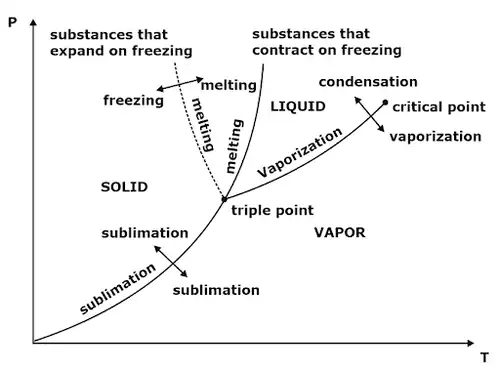Fig:3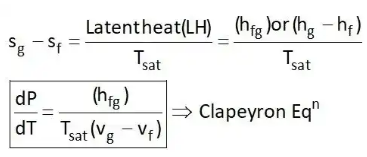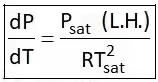The above equation is called the Clausius Clapeyron equation. It helps to determine enthalpy change associated with phase change by measuring pressure, temperature, and volume.

Offer running on EduRev: Apply code STAYHOME200 to get INR 200 off on our premium plan EduRev Infinity!

## Thermodynamics

33 videos|52 docs|36 tests

,

,

,

,

,

,

,

,

,

,

,

,

,

,

,

,

,

,

,

,

,

;